SSC CGL Previous Year Questions: Data Interpretation - 3

# SSC CGL Previous Year Questions: Data Interpretation - 3 - SSC CGL

Test Description

## 40 Questions MCQ Test SSC CGL (Tier - 1) - Previous Year Papers (Topic Wise) - SSC CGL Previous Year Questions: Data Interpretation - 3

SSC CGL Previous Year Questions: Data Interpretation - 3 for SSC CGL 2023 is part of SSC CGL (Tier - 1) - Previous Year Papers (Topic Wise) preparation. The SSC CGL Previous Year Questions: Data Interpretation - 3 questions and answers have been prepared according to the SSC CGL exam syllabus.The SSC CGL Previous Year Questions: Data Interpretation - 3 MCQs are made for SSC CGL 2023 Exam. Find important definitions, questions, notes, meanings, examples, exercises, MCQs and online tests for SSC CGL Previous Year Questions: Data Interpretation - 3 below.
Solutions of SSC CGL Previous Year Questions: Data Interpretation - 3 questions in English are available as part of our SSC CGL (Tier - 1) - Previous Year Papers (Topic Wise) for SSC CGL & SSC CGL Previous Year Questions: Data Interpretation - 3 solutions in Hindi for SSC CGL (Tier - 1) - Previous Year Papers (Topic Wise) course. Download more important topics, notes, lectures and mock test series for SSC CGL Exam by signing up for free. Attempt SSC CGL Previous Year Questions: Data Interpretation - 3 | 40 questions in 40 minutes | Mock test for SSC CGL preparation | Free important questions MCQ to study SSC CGL (Tier - 1) - Previous Year Papers (Topic Wise) for SSC CGL Exam | Download free PDF with solutions
 1 Crore+ students have signed up on EduRev. Have you?
SSC CGL Previous Year Questions: Data Interpretation - 3 - Question 1

### DIRECTIONS: Study the following table carefully to answer these questions:        (SSC CGL 2017)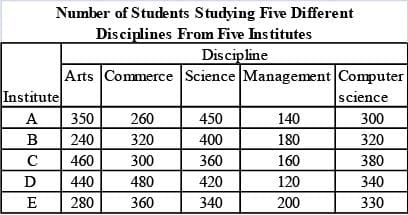Q. Total number of students studying Arts from Insitutes A and B together is approximately what per cent of the total number of students studying computer Science from these two Institutes?

Detailed Solution for SSC CGL Previous Year Questions: Data Interpretation - 3 - Question 1

Required percentage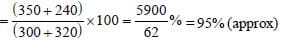SSC CGL Previous Year Questions: Data Interpretation - 3 - Question 2

### DIRECTIONS: Study the following table carefully to answer these questions:        (SSC CGL 2017)Q. What is the ratio between total number of students studying Science from Institutes C and D together and the total number of students studying Computers Science from these two Institutes together respectively?

Detailed Solution for SSC CGL Previous Year Questions: Data Interpretation - 3 - Question 2

Required ratio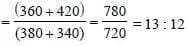SSC CGL Previous Year Questions: Data Interpretation - 3 - Question 3

### DIRECTIONS: Study the following table carefully to answer these questions:        (SSC CGL 2017)Q. What is the average number of students studying all disciplines together from institute E?

Detailed Solution for SSC CGL Previous Year Questions: Data Interpretation - 3 - Question 3

Required average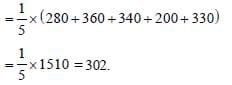SSC CGL Previous Year Questions: Data Interpretation - 3 - Question 4

DIRECTIONS: The bar graph shows the results of an annual examination in a secondary school in a certain year.      (SSC Sub Ins.  2016)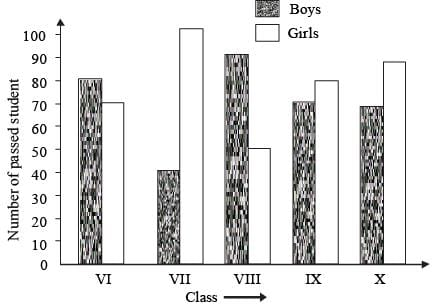Q. The ratio of the total number of boys passed to the total number of girls passed in the three classes VII, VIII and IX is

Detailed Solution for SSC CGL Previous Year Questions: Data Interpretation - 3 - Question 4

Required ratio =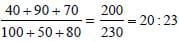SSC CGL Previous Year Questions: Data Interpretation - 3 - Question 5

DIRECTIONS: The bar graph shows the results of an annual examination in a secondary school in a certain year.      (SSC Sub Ins.  2016)Q. The average number of boys passed per class is

Detailed Solution for SSC CGL Previous Year Questions: Data Interpretation - 3 - Question 5

Average =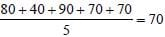SSC CGL Previous Year Questions: Data Interpretation - 3 - Question 6

DIRECTIONS: The bar graph shows the results of an annual examination in a secondary school in a certain year.      (SSC Sub Ins.  2016)Q. The class having the highest number of passed student is

Detailed Solution for SSC CGL Previous Year Questions: Data Interpretation - 3 - Question 6

The class X has the highest number of passed students.

SSC CGL Previous Year Questions: Data Interpretation - 3 - Question 7

DIRECTIONS: The bar graph shows the results of an annual examination in a secondary school in a certain year.      (SSC Sub Ins.  2016)Q. The class in which the number of boys passed is nearest to the average number of girls passed per class, is

Detailed Solution for SSC CGL Previous Year Questions: Data Interpretation - 3 - Question 7

Average number of girls =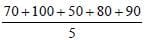= 78
The number of boys passed in class VI = 80
∴ Required answer = class VI.

SSC CGL Previous Year Questions: Data Interpretation - 3 - Question 8

DIRECTIONS: Study the following table which shows the amount of money invested (Rupees in crore) in the core infrastructure areas of two districts. A and B of a State, and answer the below five questions.         (SSC Sub Ins.  2016)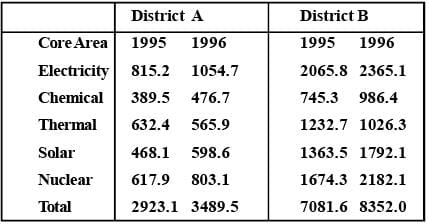Q. By approximately what percent was the total investment in the two districts A and B more in 1996 as compared to 1995?

Detailed Solution for SSC CGL Previous Year Questions: Data Interpretation - 3 - Question 8

Percentage increase =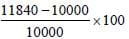= 18.4 = 18 (approx.)

SSC CGL Previous Year Questions: Data Interpretation - 3 - Question 9

DIRECTIONS: Study the following table which shows the amount of money invested (Rupees in crore) in the core infrastructure areas of two districts. A and B of a State, and answer the below five questions.         (SSC Sub Ins.  2016)Q. The total investment in electricity and thermal energy in 1995, in these two districts A and B formed approximately what percent of the total investment made in that year?

Detailed Solution for SSC CGL Previous Year Questions: Data Interpretation - 3 - Question 9

Total investment in Electricity and Thermal energy in 1995
= (815.2 + 632.4 + 2065.8 + 1232.7) = 4746.1
Percentage =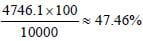SSC CGL Previous Year Questions: Data Interpretation - 3 - Question 10

DIRECTIONS: Study the following table which shows the amount of money invested (Rupees in crore) in the core infrastructure areas of two districts. A and B of a State, and answer the below five questions.         (SSC Sub Ins.  2016)Q. In district B, the investment in which area in 1996 did show the highest percentage increase over the investment in that area in 1995?

Detailed Solution for SSC CGL Previous Year Questions: Data Interpretation - 3 - Question 10

Using approximation method,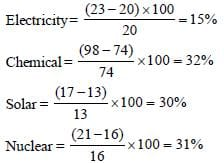∴ So, answer (c) is correct.

SSC CGL Previous Year Questions: Data Interpretation - 3 - Question 11

DIRECTIONS: Study the following table which shows the amount of money invested (Rupees in crore) in the core infrastructure areas of two districts. A and B of a State, and answer the below five questions.         (SSC Sub Ins.  2016)Q. Approximately how many times was the total investment in 1995 and 1996 in district B was that of total investment of district A in the same years?

Detailed Solution for SSC CGL Previous Year Questions: Data Interpretation - 3 - Question 11

Total investment in District A = (2923. 1 + 3489.5) = 6400 (approx)
Total investment in District B = (7081. 6 + 8352) = 15400 (approx)
Required answer = 15400/6400 = 2.4

SSC CGL Previous Year Questions: Data Interpretation - 3 - Question 12

DIRECTIONS: Study the following table which shows the amount of money invested (Rupees in crore) in the core infrastructure areas of two districts. A and B of a State, and answer the below five questions.         (SSC Sub Ins.  2016)Q. If the total investment in district B shows the same rate of increase in 1997, as it had shown from 1995 to 1996, what approximately would be the total investment in B in 1997?

Detailed Solution for SSC CGL Previous Year Questions: Data Interpretation - 3 - Question 12

% increase from 95 to 96 = % increase from 96 to 97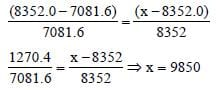SSC CGL Previous Year Questions: Data Interpretation - 3 - Question 13

DIRECTIONS: A bar graph showing the heights of six mountain peaks. Study the bar graph and answer questions.       (SSC CGL 1st Sit. 2016)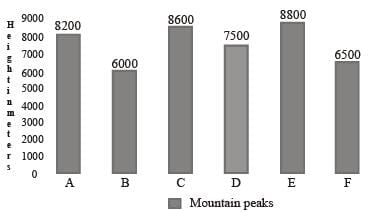Q. The average height of all the peaks (in meters) is

Detailed Solution for SSC CGL Previous Year Questions: Data Interpretation - 3 - Question 13

Average height of all the peaks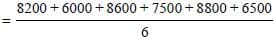= 45600/6 = 7600

SSC CGL Previous Year Questions: Data Interpretation - 3 - Question 14

DIRECTIONS: A bar graph showing the heights of six mountain peaks. Study the bar graph and answer questions.       (SSC CGL 1st Sit. 2016)Q. Which peak is the second highest?

Detailed Solution for SSC CGL Previous Year Questions: Data Interpretation - 3 - Question 14

According to bar graph, Peak of C is the second highest.

SSC CGL Previous Year Questions: Data Interpretation - 3 - Question 15

DIRECTIONS: A bar graph showing the heights of six mountain peaks. Study the bar graph and answer questions.       (SSC CGL 1st Sit. 2016)Q. Write the ratio of the heights of the highest peak and the lowest peak

Detailed Solution for SSC CGL Previous Year Questions: Data Interpretation - 3 - Question 15

Highest peak = 8800
lowest peak = 6000
Ratio = 8800/6000 = 22:15

SSC CGL Previous Year Questions: Data Interpretation - 3 - Question 16

DIRECTIONS: A bar graph showing the heights of six mountain peaks. Study the bar graph and answer questions.       (SSC CGL 1st Sit. 2016)Q. When the heights of the given peaks are written in ascending order, what is the average of the middle two peaks?

Detailed Solution for SSC CGL Previous Year Questions: Data Interpretation - 3 - Question 16

Ascending order 6000, 6500, 7500, 8200, 8600, 8800
Middle two average =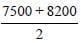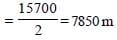SSC CGL Previous Year Questions: Data Interpretation - 3 - Question 17

DIRECTIONS: The bar graph given indicates the income of a firm. Study the graph and answer the questions given.        (SSC CGL 1st Sit. 2016)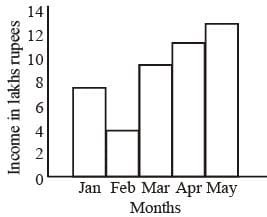Q. Which period shows a steady increase of income?

Detailed Solution for SSC CGL Previous Year Questions: Data Interpretation - 3 - Question 17

By observing the graph, we can say that study increase of income in March to May.

SSC CGL Previous Year Questions: Data Interpretation - 3 - Question 18

DIRECTIONS: The bar graph given indicates the income of a firm. Study the graph and answer the questions given.        (SSC CGL 1st Sit. 2016)Q. During which month, the ratio of the income to that of the previous month is the largest?

Detailed Solution for SSC CGL Previous Year Questions: Data Interpretation - 3 - Question 18

By observing the graph, the ratio of the income to that of the previous month is the largest on March.

SSC CGL Previous Year Questions: Data Interpretation - 3 - Question 19

DIRECTIONS: The bar graph given indicates the income of a firm. Study the graph and answer the questions given.        (SSC CGL 1st Sit. 2016)Q. The income of May is how many times to that of Februarey?

Detailed Solution for SSC CGL Previous Year Questions: Data Interpretation - 3 - Question 19

Income of February = 4
Income of May = 13
∴ Required answer = 13/4 = 3.25

SSC CGL Previous Year Questions: Data Interpretation - 3 - Question 20

DIRECTIONS: The bar graph given indicates the income of a firm. Study the graph and answer the questions given.        (SSC CGL 1st Sit. 2016)Q. The average monthly income of the firm (in lakh rupees) is

Detailed Solution for SSC CGL Previous Year Questions: Data Interpretation - 3 - Question 20

Total income of firm = 7 + 4 + 9 + 11 + 13 = 44
∴ Average monthly income = 44/5 = 8.8

SSC CGL Previous Year Questions: Data Interpretation - 3 - Question 21

DIRECTIONS: The following piechart shows the study time of different subjects of a student in a day. Study the pie chart and answer the following questions.     (SSC CGL 1st Sit. 2016)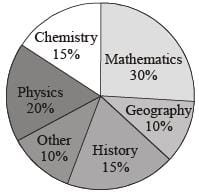Q. The time spent to study history and chemistry is 4 hours 30 minutes. Then the student studied physics for

Detailed Solution for SSC CGL Previous Year Questions: Data Interpretation - 3 - Question 21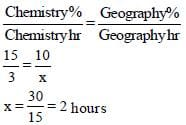SSC CGL Previous Year Questions: Data Interpretation - 3 - Question 22

DIRECTIONS: The following piechart shows the study time of different subjects of a student in a day. Study the pie chart and answer the following questions.     (SSC CGL 1st Sit. 2016)Q. If the student studied chemistry for 3 hours, then he/she studied geography for

Detailed Solution for SSC CGL Previous Year Questions: Data Interpretation - 3 - Question 22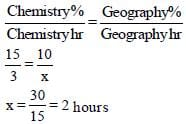SSC CGL Previous Year Questions: Data Interpretation - 3 - Question 23

DIRECTIONS: The following piechart shows the study time of different subjects of a student in a day. Study the pie chart and answer the following questions.     (SSC CGL 1st Sit. 2016)Q. If the student studied 10 hours in a day, then he/ she studied mathematics for.

Detailed Solution for SSC CGL Previous Year Questions: Data Interpretation - 3 - Question 23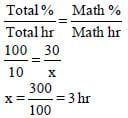SSC CGL Previous Year Questions: Data Interpretation - 3 - Question 24

DIRECTIONS: The following piechart shows the study time of different subjects of a student in a day. Study the pie chart and answer the following questions.     (SSC CGL 1st Sit. 2016)Q. Instead of 10% , if the student spends 15% to study other subjects and the time is taken from the time scheduled to study mathematics and if he/ she used to study 20 hours per day, then the difference of time for studying mathematics per day is

Detailed Solution for SSC CGL Previous Year Questions: Data Interpretation - 3 - Question 24

Other hours study = 15%
Then in Math = 30 – 5 = 25%
Difference = 5%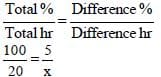x = 1 hr

SSC CGL Previous Year Questions: Data Interpretation - 3 - Question 25

DIRECTIONS: The pie–chart given here shows expenditure incurred by a family on various items and their savings. Study the chart and answer the questions based on the pie–chart.        (SSC CGL 1st Sit.  2015)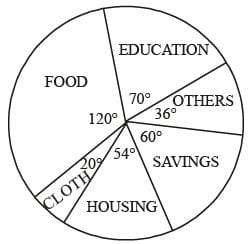Q. If the monthly income is ₹ 36000 then the yearly savings is:

Detailed Solution for SSC CGL Previous Year Questions: Data Interpretation - 3 - Question 25

Monthly savings =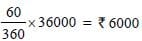Yearly savings = 6000 × 12 = ₹ 72000

SSC CGL Previous Year Questions: Data Interpretation - 3 - Question 26

DIRECTIONS: The pie–chart given here shows expenditure incurred by a family on various items and their savings. Study the chart and answer the questions based on the pie–chart.        (SSC CGL 1st Sit.  2015)Q. If the expenditure on education is ₹1600 more than that of housing then the expenditure on food is:

Detailed Solution for SSC CGL Previous Year Questions: Data Interpretation - 3 - Question 26

Let total expenditure be ₹x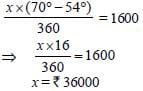∴ Expenditure on food =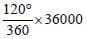= ₹ 12000

SSC CGL Previous Year Questions: Data Interpretation - 3 - Question 27

DIRECTIONS: The pie–chart given here shows expenditure incurred by a family on various items and their savings. Study the chart and answer the questions based on the pie–chart.        (SSC CGL 1st Sit.  2015)Q. The ratio of expenditure on food to savings is :

Detailed Solution for SSC CGL Previous Year Questions: Data Interpretation - 3 - Question 27

Ratio of Expenditure = 120/60 = 2/1 = 2:1.

SSC CGL Previous Year Questions: Data Interpretation - 3 - Question 28

DIRECTIONS: Study the following bar diagram carefully and answer the following four Questions.
The number of the production of electronic items (TVs and LCDs) in a factory during the period from 2009 to 2013.      (SSC CGL 1st Sit. 2015)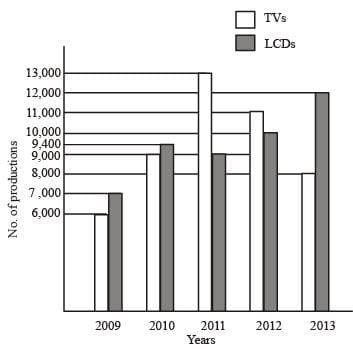Q. The total number of production of electronic items is maximum in the year

Detailed Solution for SSC CGL Previous Year Questions: Data Interpretation - 3 - Question 28

Production of electronic items is highest in 2011
i.e. 13,000 + 9,000 = 22,000

SSC CGL Previous Year Questions: Data Interpretation - 3 - Question 29

DIRECTIONS: Study the following bar diagram carefully and answer the following four Questions.
The number of the production of electronic items (TVs and LCDs) in a factory during the period from 2009 to 2013.      (SSC CGL 1st Sit. 2015)Q. The ratio of production of LCDs in the year 2011 and 2013 is

Detailed Solution for SSC CGL Previous Year Questions: Data Interpretation - 3 - Question 29

Production of LCD in 2011 = 9,000
Production of LCD in 2013 = 12,000
Ratio =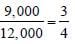SSC CGL Previous Year Questions: Data Interpretation - 3 - Question 30

DIRECTIONS: Study the following bar diagram carefully and answer the following four Questions.
The number of the production of electronic items (TVs and LCDs) in a factory during the period from 2009 to 2013.      (SSC CGL 1st Sit. 2015)Q. The difference between averages of production of TVs and LCDs from 2009 to 2012 is

Detailed Solution for SSC CGL Previous Year Questions: Data Interpretation - 3 - Question 30

Total production of TV from 2009 to 2012 = 39,000
Average production of TV = 9750
Total production of LCD = 35400
Average production of LCD = 8850
Their difference = 9,750 – 8,850 = 900

SSC CGL Previous Year Questions: Data Interpretation - 3 - Question 31

DIRECTIONS: Study the following bar diagram carefully and answer the following four Questions.
The number of the production of electronic items (TVs and LCDs) in a factory during the period from 2009 to 2013.      (SSC CGL 1st Sit. 2015)Q. The ratio of production of TVs in the years 2009 and 2010 is

Detailed Solution for SSC CGL Previous Year Questions: Data Interpretation - 3 - Question 31

Ratio of production of TV =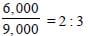SSC CGL Previous Year Questions: Data Interpretation - 3 - Question 32

DIRECTIONS: Study the following bar graph and answer the questions.        (SSC CHSL 2015)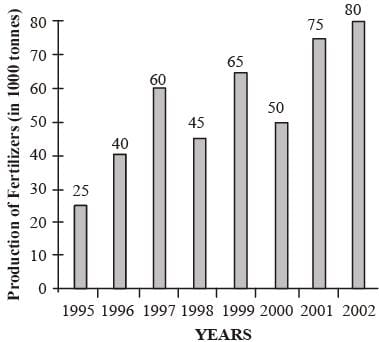Q. The number of years, the production of fertsilizers was more than average production of the given years is :

Detailed Solution for SSC CGL Previous Year Questions: Data Interpretation - 3 - Question 32

Average production of the given years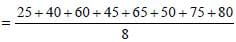= 440/8 = 55
So, during 1997, 1999, 2001 and 2002 production was more than average production.

SSC CGL Previous Year Questions: Data Interpretation - 3 - Question 33

DIRECTIONS: Study the following bar graph and answer the questions.        (SSC CHSL 2015)Q. The percentage increase in production of fertilizers in  2002 compared to that in 1995 is :

Detailed Solution for SSC CGL Previous Year Questions: Data Interpretation - 3 - Question 33

% increase in production in 2002 compared to that of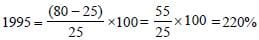SSC CGL Previous Year Questions: Data Interpretation - 3 - Question 34

DIRECTIONS: Study the following bar graph and answer the questions.        (SSC CHSL 2015)Q. The percentage decline in the production of fetilizers from 1997 to 1998 is :

Detailed Solution for SSC CGL Previous Year Questions: Data Interpretation - 3 - Question 34

% age decline in production from 1997 to 1998 is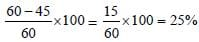SSC CGL Previous Year Questions: Data Interpretation - 3 - Question 35

DIRECTIONS: Study the following bar graph and answer the questions.        (SSC CHSL 2015)Q. The average production of 1996 and 1997 is exactly equal to the average  production of the years ?

Detailed Solution for SSC CGL Previous Year Questions: Data Interpretation - 3 - Question 35

Average production of 1996 and 1997 =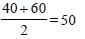Average production of years 1995 and 2001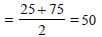So average production of 1996 and 1997 is equal to average production of 1995 and 2001.

SSC CGL Previous Year Questions: Data Interpretation - 3 - Question 36

DIRECTIONS: Study the following bar graph and answer the questions.        (SSC CHSL 2015)Q. The percentage increase in production as compared to previous year is maximum in year :

Detailed Solution for SSC CGL Previous Year Questions: Data Interpretation - 3 - Question 36

Percentage increase in production as compared to previous year is maximum in 1996 =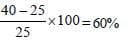SSC CGL Previous Year Questions: Data Interpretation - 3 - Question 37

DIRECTIONS: The Expenditure of a family in a month is represented by a Pie–chart. Read it and answer these questions.            (SSC CHSL 2015)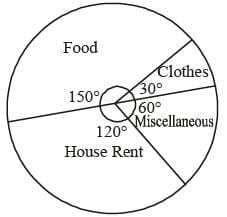Q. The ratio of the amount spent on food and clothes?

Detailed Solution for SSC CGL Previous Year Questions: Data Interpretation - 3 - Question 37

Ratio of amount spend on food and clothes
= 150 : 30
= 5 : 1

SSC CGL Previous Year Questions: Data Interpretation - 3 - Question 38

DIRECTIONS: The Expenditure of a family in a month is represented by a Pie–chart. Read it and answer these questions.            (SSC CHSL 2015)Q. The % money spent on food compared to house rent is by?

Detailed Solution for SSC CGL Previous Year Questions: Data Interpretation - 3 - Question 38

% money spent on food compared to house rent = (150/120) x 100 = 125%.

SSC CGL Previous Year Questions: Data Interpretation - 3 - Question 39

DIRECTIONS: The Expenditure of a family in a month is represented by a Pie–chart. Read it and answer these questions.            (SSC CHSL 2015)Q. The total money spent on clothes and miscellaneous items are :

Detailed Solution for SSC CGL Previous Year Questions: Data Interpretation - 3 - Question 39

Money spent on clothes and miscellaneous items cannot be determined as total money is not given.

SSC CGL Previous Year Questions: Data Interpretation - 3 - Question 40

DIRECTIONS: The Expenditure of a family in a month is represented by a Pie–chart. Read it and answer these questions.            (SSC CHSL 2015)Q. If the total amount spent is ₹ 7,200. Find the amount spent on food ?

Detailed Solution for SSC CGL Previous Year Questions: Data Interpretation - 3 - Question 40

Amount spent on food = (150/360) x 7200 = ₹3000

## SSC CGL (Tier - 1) - Previous Year Papers (Topic Wise)

250 tests
Information about SSC CGL Previous Year Questions: Data Interpretation - 3 Page
In this test you can find the Exam questions for SSC CGL Previous Year Questions: Data Interpretation - 3 solved & explained in the simplest way possible. Besides giving Questions and answers for SSC CGL Previous Year Questions: Data Interpretation - 3, EduRev gives you an ample number of Online tests for practice

250 tests